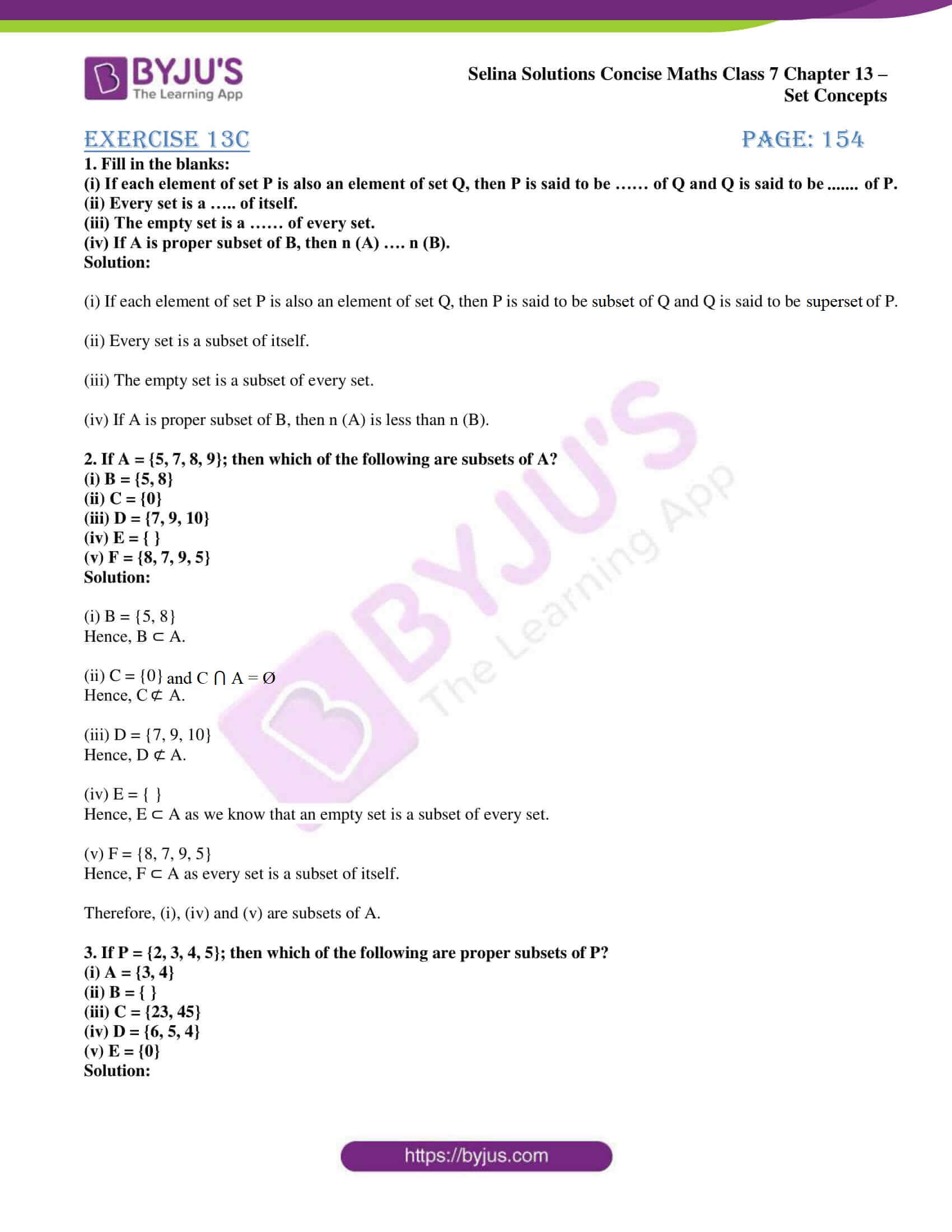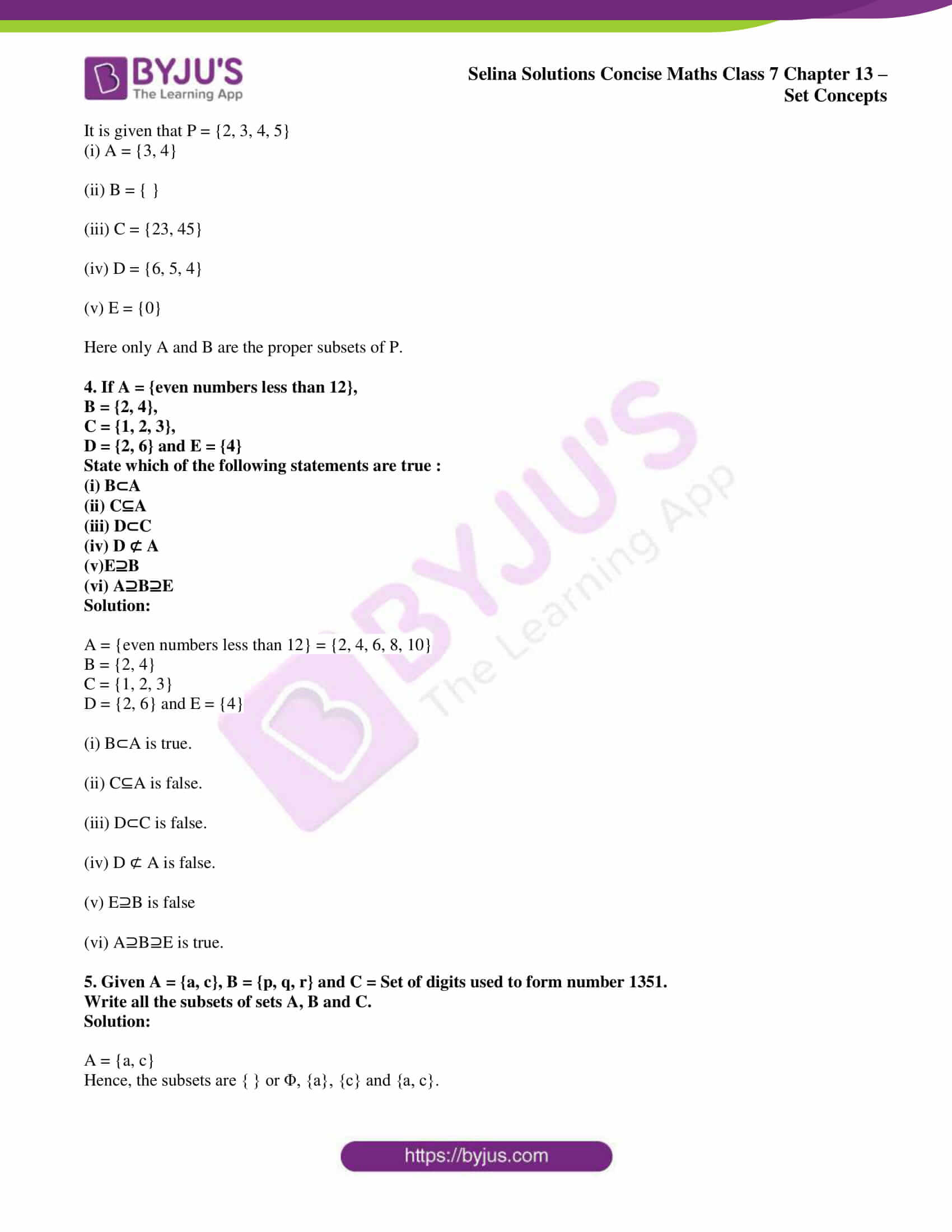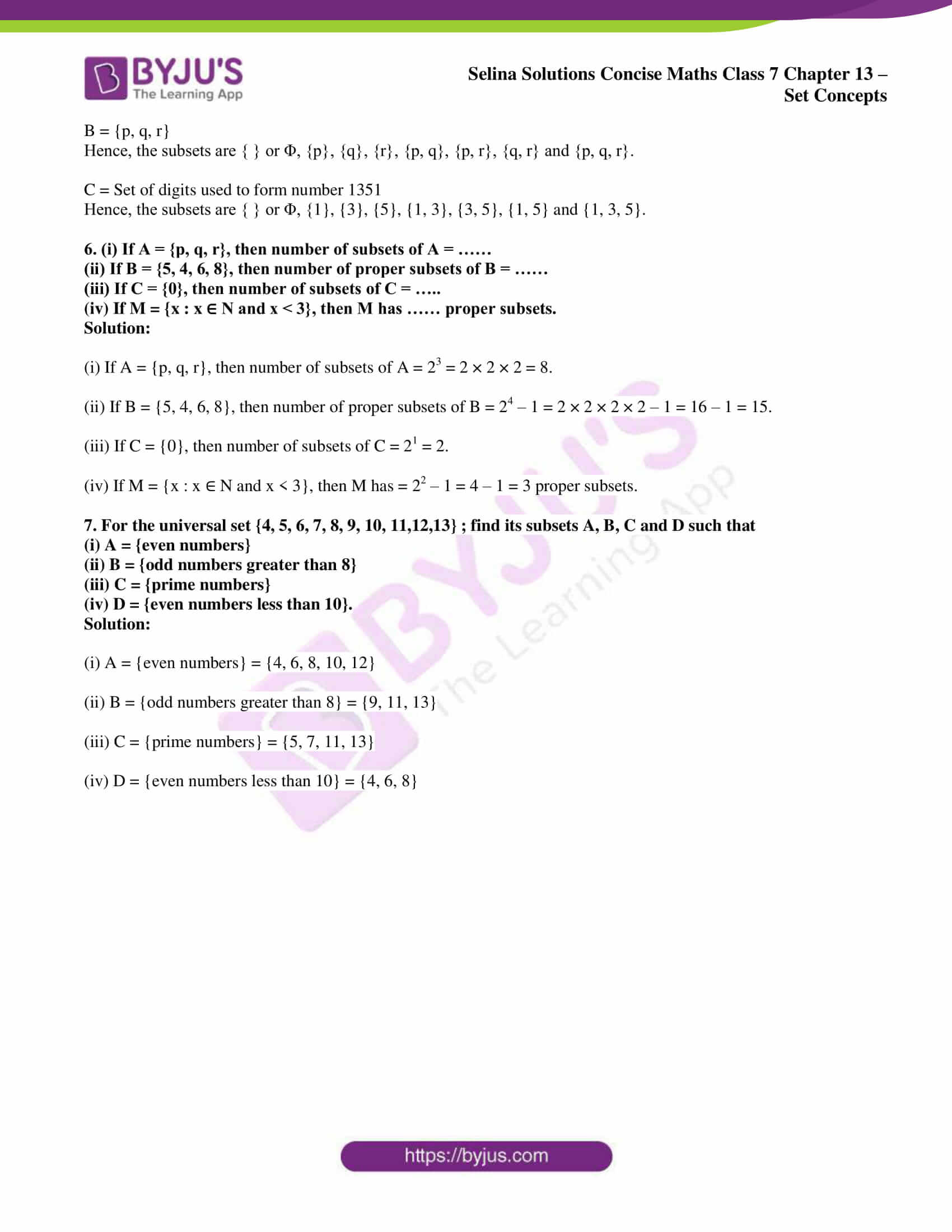# Selina Solutions Concise Maths Class 7 Chapter 13: Set Concepts Exercise 13C

Selina Solutions Concise Maths Class 7 Chapter 13 Set Concepts Exercise 13C are prepared by teachers at BYJU’S, who possess vast experience in their respective fields. The solutions are designed in an interactive way to boost interest among students in the subject. By practicing exercise wise problems, students improve their problem-solving abilities. It provides the students with a clear idea of the concepts covered in the textbook. To maintain a better academic score, Selina Solutions Concise Maths Class 7 Chapter 13 Set Concepts Exercise 13C PDF is available here, with a free download option.

## Selina Solutions Concise Maths Class 7 Chapter 13: Set Concepts Exercise 13C Download PDF### Access other exercises of Selina Solutions Concise Maths Class 7 Chapter 13: Set Concepts

Exercise 13A Solutions

Exercise 13B Solutions

Exercise 13D Solutions

### Access Selina Solutions Concise Maths Class 7 Chapter 13: Set Concepts Exercise 13C

#### Exercise 13C page: 154

1. Fill in the blanks:

(i) If each element of set P is also an element of set Q, then P is said to be …… of Q and Q is said to be ……. of P.
(ii) Every set is a ….. of itself.
(iii) The empty set is a …… of every set.
(iv) If A is proper subset of B, then n (A) …. n (B).

Solution:

(i) If each element of set P is also an element of set Q, then P is said to be a subset of Q and Q is said to be the superset of P.

(ii) Every set is a subset of itself.

(iii) The empty set is a subset of every set.

(iv) If A is proper subset of B, then n (A) is less than n (B).

2. If A = {5, 7, 8, 9}; then which of the following are subsets of A?
(i) B = {5, 8}
(ii) C = {0}
(iii) D = {7, 9, 10}
(iv) E = { }
(v) F = {8, 7, 9, 5}

Solution:

(i) B = {5, 8}

Hence, B ⊂ A.

(ii) C = {0} and C ⋂ A = Ø

Hence, C ⊄ A.

(iii) D = {7, 9, 10}

Hence, D ⊄ A.

(iv) E = { }

Hence, E ⊂ A as we know that an empty set is a subset of every set.

(v) F = {8, 7, 9, 5}

Hence, F ⊂ A as every set is a subset of itself.

Therefore, (i), (iv) and (v) are subsets of A.

3. If P = {2, 3, 4, 5}; then which of the following are proper subsets of P?
(i) A = {3, 4}
(ii) B = { }
(iii) C = {23, 45}
(iv) D = {6, 5, 4}
(v) E = {0}

Solution:

It is given that P = {2, 3, 4, 5}

(i) A = {3, 4}

(ii) B = { }

(iii) C = {23, 45}

(iv) D = {6, 5, 4}

(v) E = {0}

Here only A and B are the proper subsets of P.

4. If A = {even numbers less than 12},
B = {2, 4},
C = {1, 2, 3},
D = {2, 6} and E = {4}
State which of the following statements are true :
(i) B⊂A
(ii) C⊆A
(iii) D⊂C
(iv) D ⊄ A
(v)E⊇B
(vi) A⊇B⊇E

Solution:

A = {even numbers less than 12} = {2, 4, 6, 8, 10}

B = {2, 4}

C = {1, 2, 3}

D = {2, 6} and E = {4}

(i) B⊂A is true.

(ii) C⊆A is false.

(iii) D⊂C is false.

(iv) D ⊄ A is false.

(v) E⊇B is false

(vi) A⊇B⊇E is true.

5. Given A = {a, c}, B = {p, q, r} and C = Set of digits used to form number 1351.
Write all the subsets of sets A, B and C.

Solution:

A = {a, c}

Hence, the subsets are { } or Φ, {a}, {c} and {a, c}.

B = {p, q, r}

Hence, the subsets are { } or Φ, {p}, {q}, {r}, {p, q}, {p, r}, {q, r} and {p, q, r}.

C = Set of digits used to form number 1351

Hence, the subsets are { } or Φ, {1}, {3}, {5}, {1, 3}, {3, 5}, {1, 5} and {1, 3, 5}.

6. (i) If A = {p, q, r}, then number of subsets of A = ……
(ii) If B = {5, 4, 6, 8}, then number of proper subsets of B = ……
(iii) If C = {0}, then number of subsets of C = …..
(iv) If M = {x : x ∈ N and x < 3}, then M has …… proper subsets.

Solution:

(i) If A = {p, q, r}, then number of subsets of A = 23 = 2 × 2 × 2 = 8.

(ii) If B = {5, 4, 6, 8}, then number of proper subsets of B = 24 – 1 = 2 × 2 × 2 × 2 – 1 = 16 – 1 = 15.

(iii) If C = {0}, then number of subsets of C = 21 = 2.

(iv) If M = {x : x ∈ N and x < 3}, then M has = 22 – 1 = 4 – 1 = 3 proper subsets.

7. For the universal set {4, 5, 6, 7, 8, 9, 10, 11,12,13} ; find its subsets A, B, C and D such that
(i) A = {even numbers}
(ii) B = {odd numbers greater than 8}
(iii) C = {prime numbers}
(iv) D = {even numbers less than 10}.
Solution:

(i) A = {even numbers} = {4, 6, 8, 10, 12}

(ii) B = {odd numbers greater than 8} = {9, 11, 13}

(iii) C = {prime numbers} = {5, 7, 11, 13}

(iv) D = {even numbers less than 10} = {4, 6, 8}